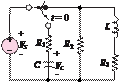### Create an Account

Already have account?

### Forgot Your Password ?

Home / Questions / At t 0 the circuit shown in Figure P566 is at steady state and the voltage across the capa...

# At t 0 the circuit shown in Figure P566 is at steady state and the voltage across the capacitor is 7 V The switch is changed as shown at t 0and

At 0, the circuit shown in Figure P5.66 is at steady state, and the voltage across the capacitor is

+7 V. The switch is changed as shown at = 0and

VS  = 12 V           = 3,300 μR1 = 9.1 kQ       R2 = 4.3 kQ R3 = 4.3 kQ     = 16 mH

Determine the initial voltage across R2 just after the switch is changed.Figure

Jun 19 2020 View more View Less

#### Answer (Solved)Subscribe To Get Solution